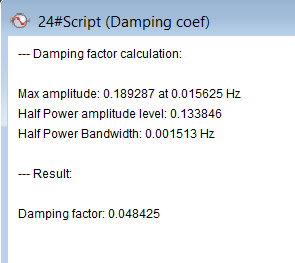Damping factor (damping ratio) is a measure of how quickly an oscillating system returns to rest after oscillating, i.e. how fast oscillations decay.

https://en.wikipedia.org/wiki/Damping

This custom tool must be used on a PSD (FFT window with PSD display type) window calculated from a vibration signal (usually acceleration signal). A dominant frequency peak must be clearly visible in the spectrum. It is recommended to calculate average of your PSD in order to get the best results.

Damping coefficient will be calculated by using a "Half Power" methodThis functions calculates the damping factor/ratio as:  ζ = Δω / 2*ωn.

The result of the analysis is displayed in a text form, for example:If a dominant frequency peak could not be determined, the damping factor will not be calculated.